RS Aggarwal Class 7 Solutions Chapter 14 - Properties Of Parallel Lines

RS Aggarwal Class 7 Chapter 14 - Properties Of Parallel Lines Solutions Free PDF

Parallel lines are lines, which do not intersect with each other and can extend indefinitely in both directions. The distance between two parallel lines is always the same and the symbol for parallel lines is ‘II’. Some of the different properties of Parallel lines are:

1. Straight lines are parallel to each other because the pair of corresponding angles are equal.
2. If the alternate angles are equal, then the two straight lines are parallel to each other.
3. The straight lines are parallel if the pair of interior angles are supplementary.

Check out the RS Aggarwal Class 7 Solutions Chapter 14 Properties of Parallel Lines.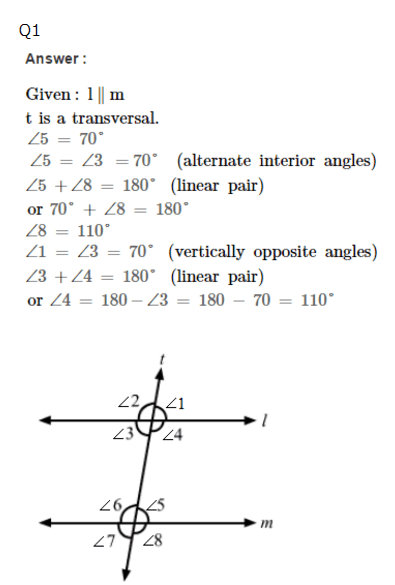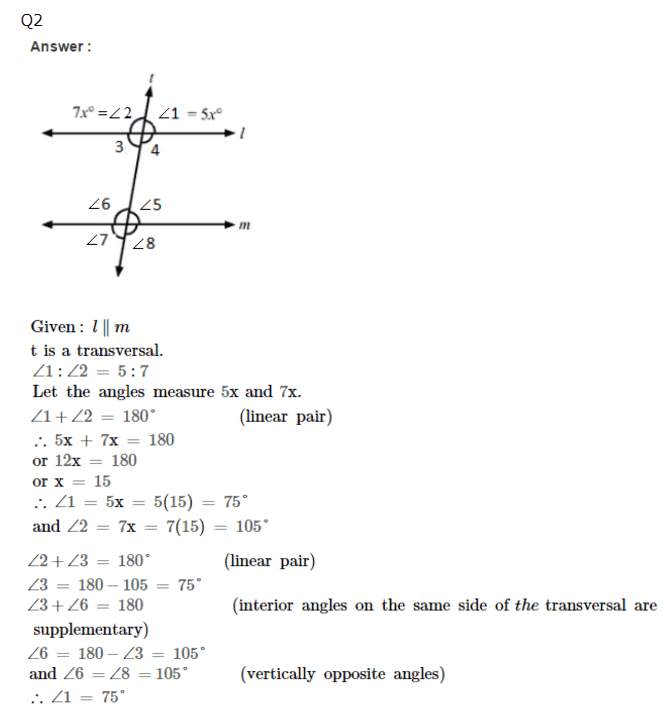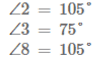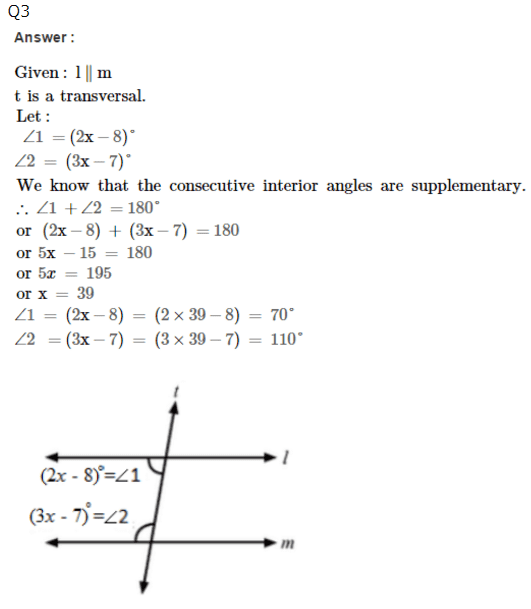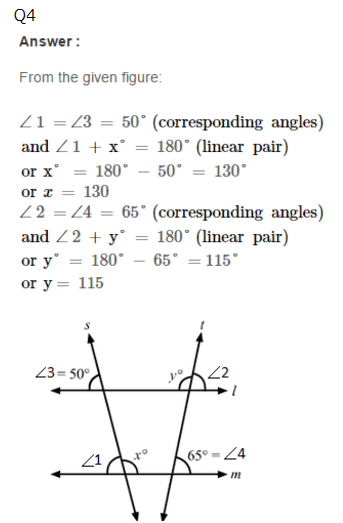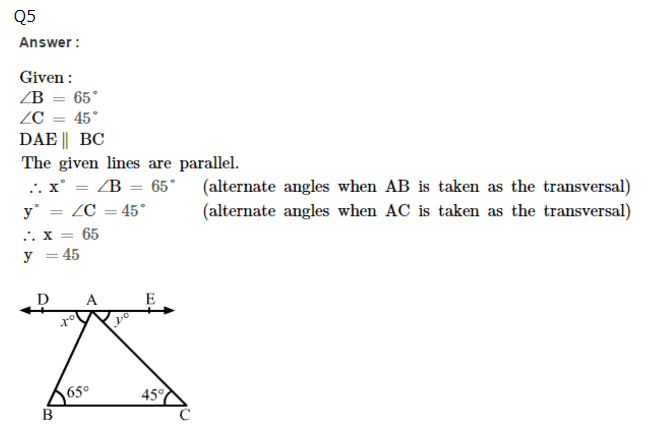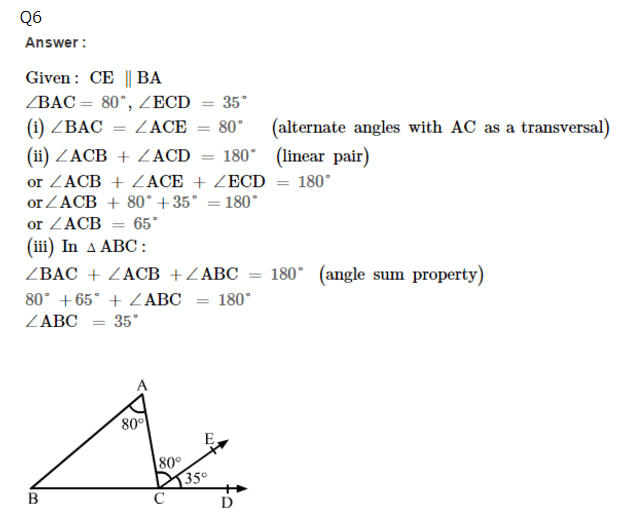Practise This Question

If 27 of 14 apples are spoilt, how many are left?